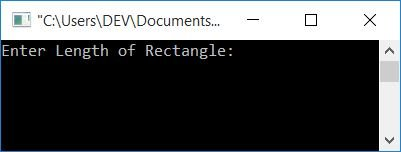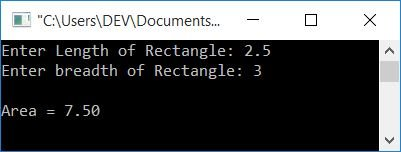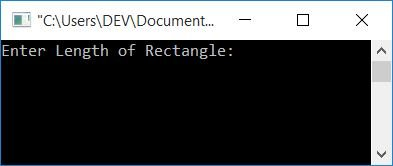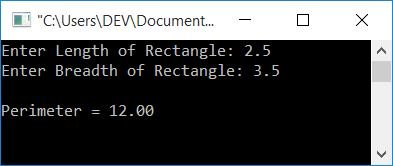# Find the Area and Perimeter of a Rectangle in C

In this article, you will learn and get code for calculating the area and perimeter of a rectangle. Here is the list of programs described in this article:

#### How to Calculate the Area of a Rectangle

To calculate the area of any rectangle, use the formula area = len*bre. Here, len is the length and bre is the breadth of the rectangle.

## Rectangle Area Calculation Program in C

The question is, "Write a program in C to calculate the area of a rectangle." The length and breadth of the rectangle must be defined by the user. The answer to this question is given below:

```#include<stdio.h>
#include<conio.h>
int main()
{
float len, bre, area;
printf("Enter Length of Rectangle: ");
scanf("%f", &len);
scanf("%f", &bre);
area = len*bre;
printf("\nArea = %0.2f", area);
getch();
return 0;
}```

This program is compiled and executed in the Code::Blocks IDE. Here is the first snapshot of a sample run produced by the above program:Now enter any input as a rectangle length, say 2.5, and press the ENTER key.Again, supply the breadth of the rectangle, say 3, and press the ENTER  key to see the output as shown in the snapshot given below:#### Steps involved in the previous program

Here is the list of some main steps used in the previous program:

• Declare any three floating-point variables.
• Here, all three variables are declared as floating-point types because they can handle real numbers or numbers that contain a decimal point.
• Now receive the length of the rectangle in the len variable.
• Again, receive the breadth of the rectangle in the bre variable.
• Apply the formula to calculate the area of a rectangle, that is, len*bre, and initialize it to the area variable.
• Print the value of the area variable that shows the area of the rectangle.
• Here, the format specifier %0.2f is used to print the value of the area variable up to to 2 decimal places only.

## Area of Rectangle Program in C Using Function

Let's create another program that does the same job as the previous program. The only difference is that the previous program found the area of a rectangle without using a function, but this program uses a user-defined function to find the area of a rectangle as given below:

```#include<stdio.h>
#include<conio.h>
float findAreaFun(float, float);
int main()
{
float len, bre, area;
printf("Enter Length of Rectangle: ");
scanf("%f", &len);
scanf("%f", &bre);
area = findAreaFun(len, bre);
printf("\nArea = %0.2f", area);
getch();
return 0;
}
float findAreaFun(float l, float b)
{
return l*b;
}```

This program produces the same output as the previous program.

#### How to Calculate the Perimeter of a Rectangle

To calculate the perimeter of a rectangle, use the formula perimeter = 2*(len+bre). Here, len indicates the length and bre indicates the breadth of the rectangle.

A rectangle's perimeter is the length of its boundary. And there are four boundaries of a rectangle, in which the two adjacent boundaries are equal to their opposite boundaries. To calculate perimeter, we can also write the above formula as perimeter = len+len+bre+bre.

## Perimeter of the Rectangle Program in C

The question is: write a program in C that prints the perimeter of a rectangle. The user must specify the length and width of the rectangle. The answer to this question is given below:

```#include<stdio.h>
#include<conio.h>
int main()
{
float len, bre, perimeter;
printf("Enter Length of Rectangle: ");
scanf("%f", &len);
scanf("%f", &bre);
perimeter = 2*(len+bre);
printf("\nPerimeter = %0.2f", perimeter);
getch();
return 0;
}```

Here is a sample run of the above program:Now, one by one, supply any two inputs as the length and breadth of a rectangle, say 2.5 (as length) and 3.5 (as breadth). Press the ENTER key to see the output as shown in the snapshot given below:## Rectangle Perimeter in C Using Function

Here is the last program of this article. This program does the same job as the previous program. The only difference in coding for this program is that it is built around a function that calculates the perimeter of a rectangle. Consider the following:

```#include<stdio.h>
#include<conio.h>
float findPerimeterFun(float, float);
int main()
{
float len, bre, perimeter;
printf("Enter Length of Rectangle: ");
scanf("%f", &len);
scanf("%f", &bre);
perimeter = findPerimeterFun(len, bre);
printf("\nPerimeter = %0.2f", perimeter);
getch();
return 0;
}
float findPerimeterFun(float l, float b)
{
return 2*(l+b);
}```

The output of the preceding program is identical to that of the previous program.

C Quiz

« Previous Program Next Program »